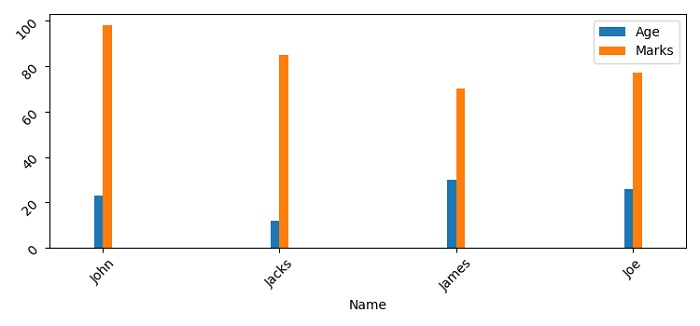# Setting the spacing between grouped bar plots in Matplotlib

To set the spacing between grouped bar plots in matplotlib, we can take the following steps −

• Set the figure size and adjust the padding between and around the subplots.

• Create a dictionary for bar details to be plotted.

• Make a Pandas dataframe using dictionary, d.

• Plot the bar using dictionary, d, with align="center".

• To display the figure, use show() method.

## Example

import matplotlib.pyplot as plt
import pandas as pd

plt.rcParams["figure.figsize"] = [7.50, 3.50]
plt.rcParams["figure.autolayout"] = True

d = {"Name": ["John", "Jacks", "James", "Joe"],"Age": [23, 12, 30, 26],"Marks": [98, 85, 70, 77]}

df = pd.DataFrame(d)
df.set_index('Name').plot(kind="bar", align='center', width=0.1)
plt.tick_params(rotation=45)

plt.show()

## OutputUpdated on: 03-Jun-2021

2K+ Views